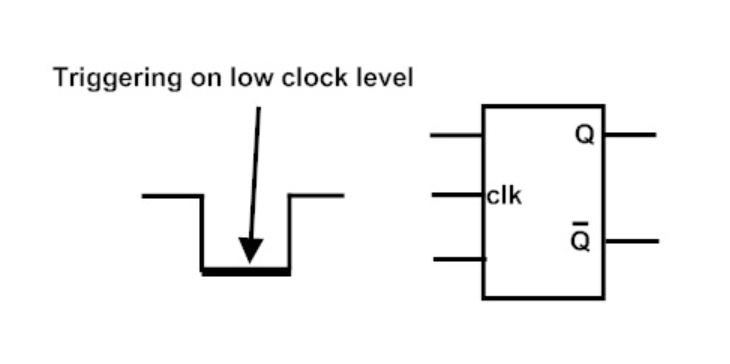In this article, we will be discussing sequential logic circuits and more related topics.

As we can see in the block diagram given below, a sequential logic circuit consists of a combinational circuit and storage element, it not only depends on the present values of input but also on the previous values of inputs.Therefore to store the previous values, a storage element is included, it can store binary information which is given at any time. Examples of sequential circuits are latches, flip flops, counters etc.

As you got a rough idea about sequential circuits, let’s look into the differences between combinational circuits and sequential circuits to get a clear idea.

## Types of sequential circuits

There are two types of sequential circuits: Asynchronous and synchronous sequential circuits.

Asynchronous sequential circuit

Asynchronous sequential circuits are driven by the pulses of the inputs instead of using the clock signals, that is the state of circuit changes when the inputs change. The change in internal state occurs when there is change in the input variable. Un-clocked flip flops or time delay elements are used as memory elements.

Synchronous sequential circuit

In synchronous sequential circuits, the state of memory changes with the clock signal connected. That is, all the outputs are affected by the clock signal at the same time. Here, flip flops and latches are used as memory elements.

As you have noticed clock signals and pulses, now let’s discuss the clock and its related topics.

## Clock and Triggering

Clock

The clock signal has an important part in the sequential circuits. A clock oscillates between 0 and 1, that is between ON and OFF. It can be represented using square wave form, when both ON and OFF are not the same. These signals control how and when the output of the sequential circuits changes.

## Types of triggering

There are two types of triggering – edge triggering and level triggering.

Edge triggering

In this, the output changes when a transition occurs, that is when logic changes from 0 to 1 or from 1 to 0.

• Positive edge triggering

Here the circuit activates, when the transition occurs from logic 0 to logic 1.

Negative edge triggering

Here the circuit activates when the transition occurs from logic 1 to logic 0.

Level triggering

In this, there are two levels – logic high and logic low. A circuit is activated only when the clock pulse is at a particular level.

• High level triggering

In this triggering, the circuit operates only when the signal is logic high or logic 1.

Low level triggering

In this triggering, the circuit operates only when the signal is logic low or logic 0.## Latches

Latches are the storage elements that operate with signal levels, and are said to be level sensitive devices. These are used for storing binary information using feedback and for designing asynchronous sequential circuits. Latches are related to flip flops, as latches are the basic circuits from which flip flops are constructed and hence, they are not practical for use in synchronous sequential circuits. Examples are SR latch,D latch etc.

SR latch

## Flipflops

Flip flops are basic storage elements similar to latches which are the fundamental building blocks of sequential circuits. A circuit that has a feedback path and two stable states which store binary data by applying different inputs are treated as flip flops.

We can directly implement flip flops or by cascading two latches. Flip flops are edge sensitive, that is it is operated in the transition from logic 0 to 1 or from logic 1 to 0.

Examples are SR, JK, T, D flip flop etc.

SR flip flop